1.0 Fun with Numbers:

Before we study numbers and their properties, let us familiarise few interesting things about numbers

Telling a number:

Can you tell a number a friend has in his mind without him revealing that number to you?

Here are the steps

 Step No Action Example 1 Ask your friend to keep a number in his mind Say 27 2 Ask him to multiply that number by 2 54 3 Ask him to add 4 to that 58 4 Ask him to divide the number by 2 29 5 Ask him to reveal the number to you 29

Now you subtract 2 from that number and tell him that his number is 27!

What is the mathematics behind that?

Let x be the number.

 Step No Action Example 1 Ask your friend to keep a number in his mind Say x 2 Ask him to multiply that number by 2 2x 3 Ask him to add 4 to that 2x+4 4 Ask him to divide the number by 2 x+2 5 Ask him to reveal the number to you x+2

When you subtract 2 from that you get x which is the number he had in his mind

Can you construct a different method by using different multiplication factor in stead of 2 and also different additional factor instead of 4?

There are innumerable numbers of ways, we can formulate above type of tricks/puzzles.

Let us now look at some interesting facts about some numbers.

Speciality of numbers from 1 to 9:

1.   Consider the number 123456789. Sum of individual digits of this number is 45(=1+2+3+4+5+6+7+8+9)

2.   Now multiply 123456789 by 2 and we get 246913578. Again sum of individual digits of this number is also 45(=2+4+6+9+1+3+5+7+8) Also note that no digit repeats in the result and no digit is missing between 1 and 9. What do you observe when 123456789 is multiplied by 4,5,7,8?

3.   Let us look at the multiples of 9: They are 9, 18, 27, 36, 45, 54, 63, 72, 81, 90,99,108,117..( If we add the individual digits of these multiples we get the sum as 9(1+8=9,2+7=9.. )

Properties of squares: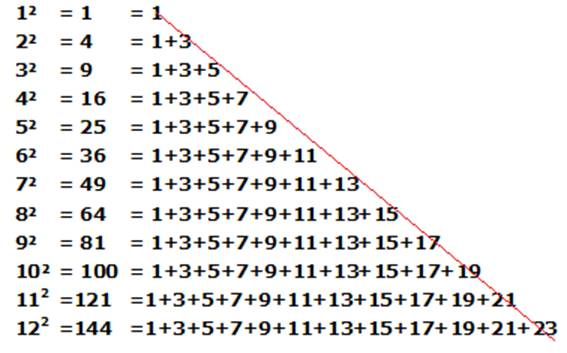What did we notice?

The result of squaring a number can also be arrived at by progressively adding consecutive odd numbers as shown above

Is this surprising? There is mathematics behind it if we know the formula for formula for (a+b)2 which we learn later in algebra

Let us look at the expansion for (n+1)2 for any number.

We learn later that (n+1)2= n2+2n+12= n2+(2n+1).

Note here that here 2n+1 is a consecutive odd number after the odd number in n2

Meru Prastara: Observe the following arrangement of numbers: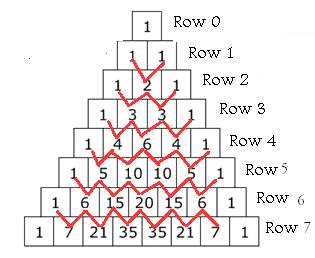In this triangle type of figure, at the tip is a small square containing the number 1, which makes up the 0th row. In each row, value at the extreme ends of the row on both sides are said to have value 0. The first row (1 & 1) contains two 1's, both formed by adding the two numbers above them to the left and the right, in this case 1 and 0. Do the same to create the 2nd row: 0+1=1; 1+1=2; 1+0=1. And the third: 0+1=1; 1+2=3; 2+1=3; 1+0=1. In this way, the rows of the triangle go on forever. A number in the triangle can also be found by nCr (n Choose r) where n is the number of the row and r is the order of element in the square corresponding to that row. For example, in row 3, 1 is the 0th element, 3 is first element, the next 3 is the 2nd element, and the last 1 is the 3rd element. The formula for nCr is: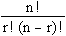(We learn the concept in the chapter on Permutations and Combinations later in a higher class)

First reference to this type of arrangement was formed by Pingala(Period : 2nd BC). He used this concept in Chandas Shastra( Study of syllables). It was called Meru Prastara( Steps of Mountain Meru ). Halayudha a 10th Century Mathematician provided commentary on this arrangement. As is the case with crediting all the discoveries of Indian mathamaticians after westerners, this triangle is now called Pascal triangle named after Pascal(17th Century) mach after its discovery and relevance 1900 years ago!

Now lets us look at the some of the properties of this Pingalas Meru Prastara.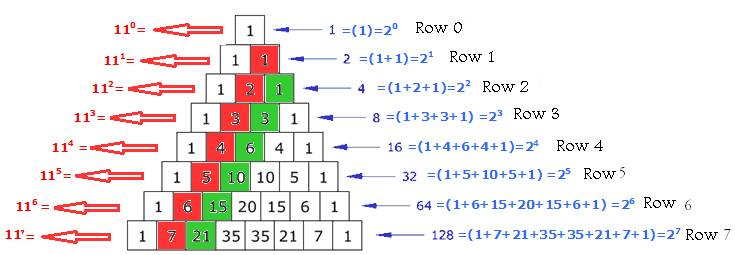Properties: Let n be the row number starting from 0

1.   Sum of the numbers across a horizontal row is always 2n .( Ex 1 = 20 1+1=2=21, 1+2+1=4= 22 1+3+3+1=8=23,, Denoted by line marked as blue)

2.   The numbers when arranged across a horizontal row represents 11n ( Ex: 1= 110,11 = 111 ,121= 112, 1331= 113Denoted by line marked as red )

3.   The numbers across a diagonal represent natural numbers (1,2,3. Denoted by squares in red colour)

4.   The numbers across a diagonal when added with previous numbers is a perfect square (1= 12, 1+3=4=22, 3+6=9=32, 6+10=16= 42,. Denoted by squares in green colour)

(Perfect square is a number made by squaring a whole number)

5.   If the 2nd element in a row is prime number other than 2, then all next numbers in that row are multiples of it( 3, 3 in Row 3, 5,10,10,5 in Row5, 7,21,35,35,21,7 in Row 7)

Let us look at the above arrangement from a different perspective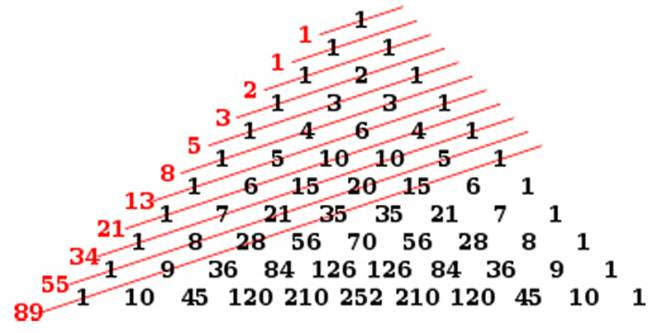The numbers indicated in red are the sum of numbers in diagonals. They are

1 1 2 3 5 8 13 21 34 55 89

Except for first number 1, each of the subsequent number is sum of previous 2 numbers (13=5+8, 21=8+13, 34=13+21..)

## The above series is now called Fibonacci Series named after 12th century mathematician Fibonacci.

Let us now look at another interesting mathematics concept called Magic square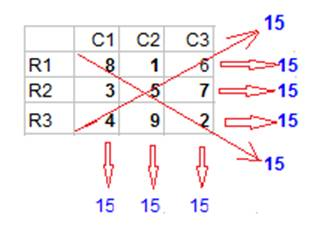What do we observe in the figure? The figure has 3 rows (R1, R2 and R3). It also has 3 columns (C1, C2 and C3) and in all has 9 squares (3*3). Squares are filled with numbers from 1to 9 without any number repeating and without any number missing from 1 to 9. We also observe

Sum of numbers in every Row (R1, R2 and R3) is 15

Sum of numbers in every column (C1, C2 and C3) is 15

Sum of numbers across 2 diagonals (joined by red line) is also 15.

15 is called magic sum. Magic sum is the sum of all numbers in any row/column/diagonal in a magic square.

Observe the below mentioned figure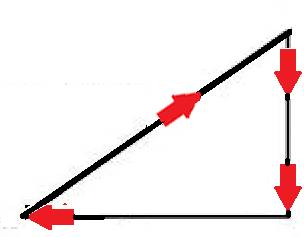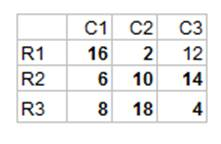Above is another magic square whose magic sum is 30 and squares have all even numbers without any repetition and without any missing number starting from 2 to 18.(In all 9 even numbers)

Construction of magic square is very simple if you can remember the right angled triangle

For easy remembrance rule can be simply stated as Diagonal, Down, Left

Rules:

Start with placing the number in the top row and middle column in an odd numbered square, and next number in the diagonal square in the top

 Rule No Action 1 If there is no row and no column for a number then place the number immediately below it else place it in the bottom most square in the same column 2 If the square is already occupied, then place it immediately below in the same column 3 If there is no column then place the number in extreme left square in the same row

Let us see now how to use the above rules to construct the Magic square given below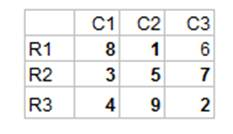Step No Action(Row R0 and Column CL are only for demonstration) Movement (Numbers in row R0 and Column CL have numbers which we place diagonally before they are moved correctly in the magic square) 1 Start with number 1 in middle row, middle column(R1,C2)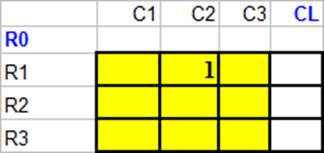2 Try to place next number 2 diagonally above, Since there is no row for that number, but since there is column for that number, place it in the bottom most square in the same column (R3,C3) Rule 1 else Condition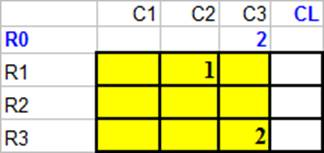3 Try to place next number 3 diagonally above, Since there is no column for that number, place it in the extreme left square in the same row(R2,C1) Rule 3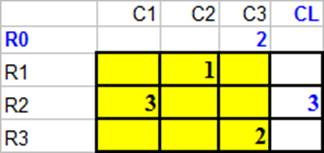4 Try to place next number 4 diagonally above(R1,C2), Since that square is already occupied , place it in the square below in the same column (R3,C1) Rule 2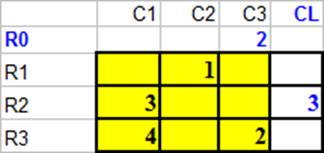5 Place next 2 numbers diagonally. 5 in (R2,C2), 6 in (R1,C3)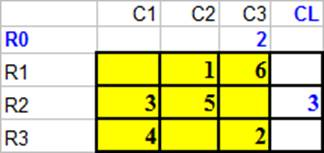6 Try to place next number 7 diagonally above, Since there is no row and no column for that number, place it just below 6. (R2,C3) Rule 1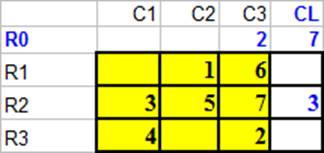7 Try to place next number 8 diagonally above, Since there is no column for that number, place it in the extreme left square in the same row(R1,C1) Rule 3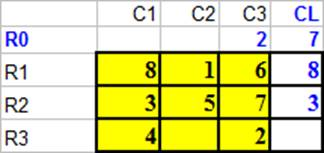8 Try to place next number 9 diagonally above, Since there is no row for that number, but since there is column for that number, place it in the bottom most square in the same column (R3,C2) Rule 1 else Condition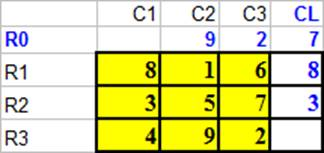Thus with just 3 easy rules and Diagonal, Down, Left Concept we are able to create a 3 by 3 Magic square

Similarly one can create 5 by 5 and 7 by 7 Magic square an example of which is given below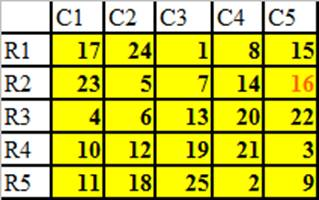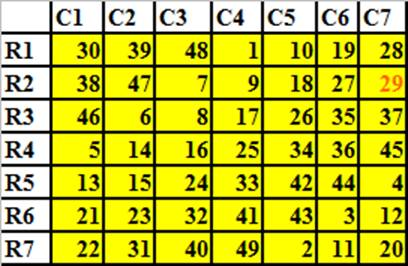In the above examples, 16 and 29 are marked in red to indicate that only these 2 numbers do not follow the else part in rule 1.

Now construct a 7 by 7 magic square with odd numbers starting from 1 using the rules stated above as follows: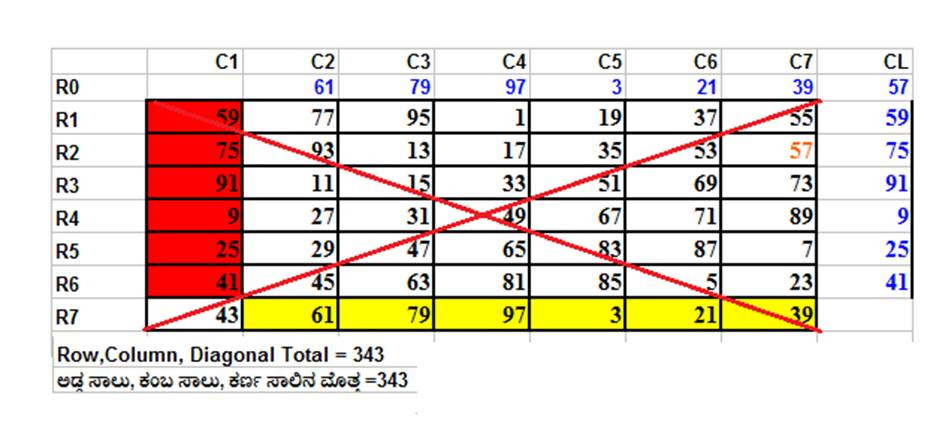Construction of magic square with magic sum of any given number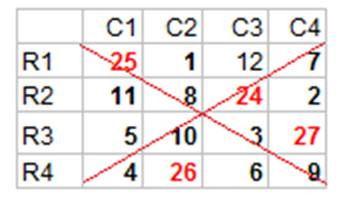In the above figure note that sum of numbers in 4 rows(R,,R4), sum of numbers in 4 columns(C1,,C4) and sum of numbers in 2 diagonals(crossed by red line) are all same and is equal to 45.

How do we construct such magic squares?

Let x be the given Magic sum for which we need to construct the magic square. We use 9 in 4 different ways to fill the some of the squares in a 4 by 4 square.

 Step/Figure Action Movement 1 Note 9= 5+4, use these 2 numbers (5 and 4) to fill the squares in the squares of last 2 rows(R3 and R4) in the first column(C1)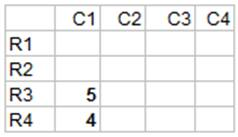2 Note 9=1+8, use these 2 numbers(1 and 8) to fill the squares in the squares of first 2 rows(R1 and R2) in the second column(C2)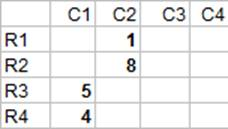3 Note 9= 3+6, use these 2 numbers (3 and 6)to fill the squares in the squares of last 2 rows(R3 and R4) in the third column(C3)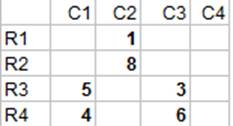4 Note 9=7+2, use these 2 numbers(7and 2) to fill the squares in the squares of first 2 rows(R1 and R2) in the fourth column(C4)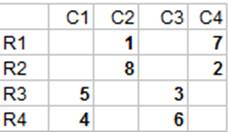5 Place 9 in the last row(R4) of last column(C4)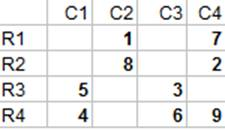6 Place 10, 11, 12 in the squares in (R3,C2),(R2,C1) and (R1,C3) respectively as shown in the figure 6.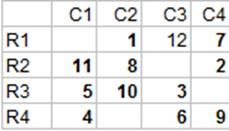7 If the magic sum required is X, then place X-20,X-19,X-21,X-18 in (R1,C1), (R4,C2),(R2,C3) and (R3,C4) respectively as shown in the figure 7.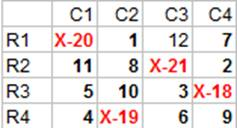Note the following in the figure/step number 7.

·       Sum of numbers in Row R1= X-20+1+12+7= X

·       Sum of numbers in Column C4 = 7+2+X-18+9=X

·       Sum of numbers in the diagonal top to bottom= X-20+8+3+9=X

·       Similarly one can observe that sum of numbers in other rows, other columns and another diagonal is X

If we want a magic square whose magic sum required is 45, then substitute 45 for x in the last figure to get the desired magic square as in figure 8 which is what we started with as an example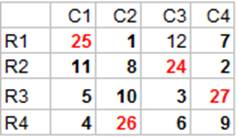Having seen and understood some of interesting facts about numbers, let us learn about divisibility tips which are useful in case of simplification:

 Divisible by: If: Examples: 2 The last digit is even (0,2,4,6,8) 128 Yes   129 No 3 The sum of the digits is divisible by 3 381 (3+8+1=12, and 12������ 4) Yes   217 (2+1+7=10, and 10������ 3 1/3) No 4 The number formed by last 2 digits is divisible by 4 1312 Yes (12������) 7019 No 5 The last digit is 0 or 5 175 Yes 809 No 6 The number is divisible by both 2 and 3 114 (it is even, and 1+1+4=6 and 6������ 2) Yes 308 (it is even, but 3+0+8=11 and 11������ 3 2/3) No 7 If you double the last digit and subtract it from the rest of the number and the answer is: ·                     0, or ·                     divisible by 7 (Note: you can apply this rule to that answer again if you want) 672 (Double of 2 is 4, 67-4=63, and 63������) Yes   905 (Double of 5 is 10, 90-10=80, and 80������1 3/7) No 8 The number formed by last three digits is divisible by 8 109816 (816������02) Yes   216302 (302������7 3/4) No 9 The sum of the digits is divisible by 9 (Note: you can apply this rule to that answer again if you want) 1629 (1+6+2+9=18, and again, 1+8=9) Yes   2013 (2+0+1+3=6) No 10 The number ends in 0 220 Yes 221 No 11 If (sum of digits in even paces- sum of digits in odd places)= ·                     0, or ·                     divisible by 11 1364 ((3+4) - (1+6) = 0) Yes 3729 ((7+9) - (3+2) = 11) Yes   25176 ((5+7) - (2+1+6) = 3) No 12 The number is divisible by both 3 and 4 648 (By 3? 6+4+8=18 and 18������ Yes By 4? 48������2 Yes) Yes 524 (By 3? 5+2+4=11, 11������3 2/3 No Don't need to check by 4.) No

1.1 Summary of learning

 NO Points studied 1 Meru Prastara Different types of majic squares 2 Divisibility test